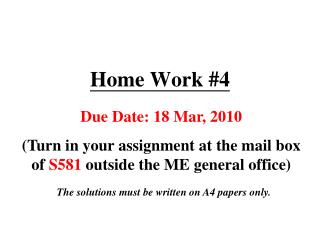DownloadDownload PresentationHome Work #4

# Home Work #4

Télécharger la présentation## Home Work #4

- - - - - - - - - - - - - - - - - - - - - - - - - - - E N D - - - - - - - - - - - - - - - - - - - - - - - - - - -
##### Presentation Transcript

1. Home Work #4 Due Date: 18 Mar, 2010 (Turn in your assignment at the mail box of S581 outside the ME general office) The solutions must be written on A4 papers only.

2. HW 4-Problem #1 A flat bar of rectangular cross section, length L, and constant thickness t is subjected to tension by forces P. The width of the bar varies linearly from b1 at the smaller end to b2 at the larger end. Assume that the angle of taper is small. Derive the following formula for the elongation of the bar: Calculate the elongation, assuming L=5 ft, t= 1.0 in., P=25 k, b1=4.0 in., b2=6.0 in., and E=30×106 psi.

3. HW 4-Problem #2 A rigid bar of weight W=800 N hangs from three equally spaced vertical wires, two of steel and one of aluminum. The wires also support a load P acting at the midpoint of the bar. The diameter of the steel wires is 2 mm, and the diameter of the aluminum wire is 4 mm. What load Pallow can be support if the allowable stress in the steel wires is 220 MPa and in the aluminum wire is 80 Mpa?

4. HW 4-Problem #3 A copper bar AB of length 25 in. is placed in position at room temperature with a gap of 0.008 in. between end A and a rigid restraint. Calculate the axial compressive stress σc in the bar if the temperature rises 50ºF. For copper, use α=9.6×10-6 /ºF and E=16×106 psi.

5. HW 4-Problem #4 A tension member is to be constructed of two pieces of plastic glued along plane pq. For purpose of cutting and gluing, the angle θ must be between 25˚ and 45˚. The allowable stresses on the glued joint in tension and shear are 5.0 Mpa and 3.0 Mpa, respectively. Determine the angle θ so that the bar will carry the largest load P. (Assume that the strength of the glued joint controls the design.) Determine the maximum allowable load Pmax if the cross-sectional area of the bar is 225 mm2.